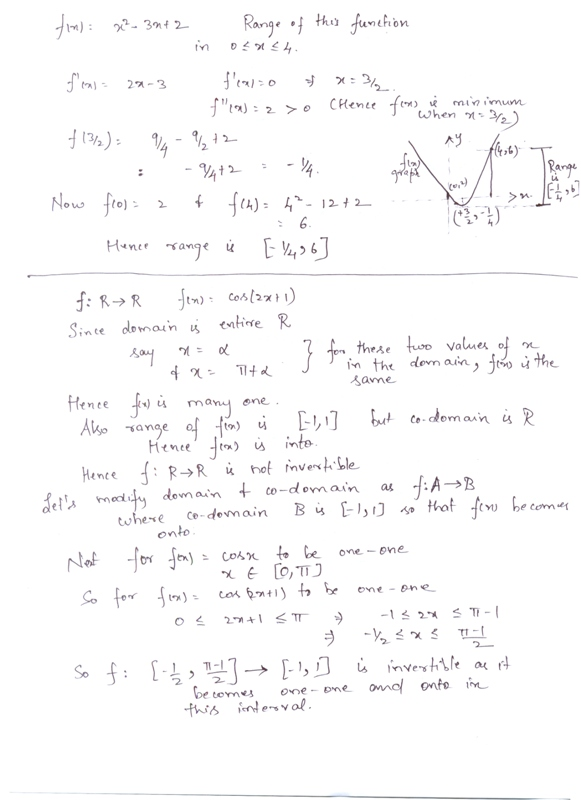# Is the function f:R→R defined by f(x)=cos(2x+1) invertible?Give reasons.Can you modify domain and codomain of f such that f becomes invertible?11 years ago

Dear Menka,

no the function is not invertible as the function f(x) is not BIJECTIVE as it is not one to one

as there are many values of x for which there exist values of -1 to 1 the range of the function

and the function is also not onto as the codomain is all real number R but the range of f(x) is -1 to 1

and for onto the codomain must be equal to range .

as we know the necessary and sufficient condition for the function to be invertible should be

that the function must be bijective .

to make function invertible the codomain must be equal to [-1 , 1]

and domain must be

0 <= 2x + 1 <= pie

now by this relation we get

-1/2 <= x <= (pie - 1 )/2

so the domain must be equal to -1/2 <= x <= (pie - 1 )/2

and codomain is  [-1 , 1]. to make function invertible

Now you can win exciting gifts by answering the questions on Discussion Forum. So help discuss any query on askiitians forum and become an Elite Expert League askiitian.

jitender

11 years ago

Hi Menka,

Here's the answer in the scanned copy:Hope that helps.

All the best.

Regards,